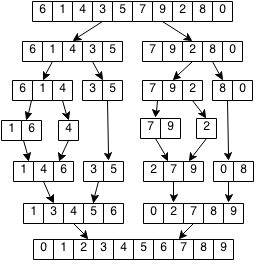## Introduction

Prerequisites: Recursion

Merge sort works by breaking down the sorting into smaller pieces. If we want to sort N elements, we can sort the first half of the elements, sort the second half and then merge the results together. To sort the first half, we can do the exact same thing of sorting the first quarter and the second quarter and merging the results.

## Implementation

Merge sort work be breaking down the problem into smaller and smaller parts and then combining those parts to solve the larger problem.

We can keep splitting the list into half until each piece has zero or one elements. We can then combine the results of each piece repeatedly until the entire list is sorted.

Example:### Formalization

```Let merge(arr1,arr2) that combines two sorted arrays into one sorted array.

Example:
merge([1,5,7,9], [2,4,8])
= [1, 2, 4, 5, 7, 8, 9]

Let sort(arr) sort an array
Let middle be arr.length / 2

sort([x]) = [x]
sort([x,y]) = [x,y] if x<y
sort([x,y]) = [y,x] otherwise
sort(arr) = merge(sort(arr[0..middle]), sort(arr[middle..arr.length])

Example:
sort([3, 7, 1, 9, 8, 4, 5])
= merge(sort([3, 7, 1]), sort([9, 8, 4, 5]))
= merge(merge(sort([3, 7]), sort(), merge(sort([9, 8]), sort([4, 5])))
= merge(merge([3, 7], ), merge([8, 9], [4, 5])
= merge([1, 3, 7], [4, 5, 8, 9])
= [1, 3, 4, 5, 7, 8, 9]
```

### Code

We will implement our solution in Java that reflects our formalization in a way that is easier to understand but more inefficient. In our code, we create new arrays to store merged results but we can actually do this in place without extra memory (left as an exercise).

```// Merges two sorted arrays v1 and v2 into a new sorted array
public static Vector<Integer> merge(Vector<Integer> v1, Vector<Integer> v2) {
Vector<Integer> merged = new Vector<Integer>();
int i = 0, j = 0;
// Always take the smaller element of the two vectors
while(i < v1.size() && j < v2.size()){
if(v1.get(i) < v2.get(j)){
i++;
} else {
j++;
}
}
if (i >= v1.size()){
// Add the rest of v2
while(j < v2.size()){
j++;
}
} else {
// Add the rest of v1.
while(i < v1.size()){
i++;
}
}
return merged;
}

// Merge sorts an array
public static Vector<Integer> mergeSort(Vector<Integer> v) {
// Base case if 1 or 0 elements.
if (v.size() <= 1) {
return v;
}
// Get middle of array.
int middle = v.size()/2;
// Split vector into two halves.
Vector<Integer> firstHalf = new Vector<Integer>(v.subList(0, middle));
Vector<Integer> secondHalf = new Vector<Integer>(v.subList(middle, v.size()));
// Return merged halves.
return merge(mergeSort(firstHalf), mergeSort(secondHalf));
}

// Example usage:
Vector<Integer> v = new Vector<Integer>();